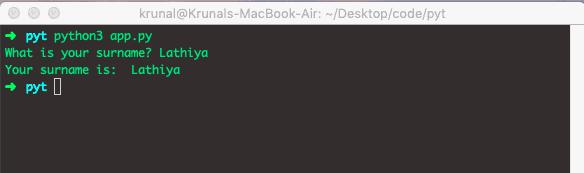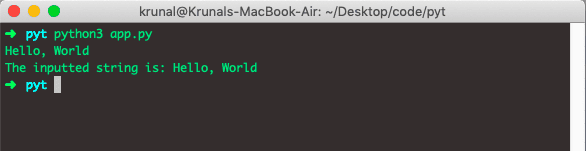# Python input from user: The Complete Guide

Developers often need to interact with users to get data or provide results. Most programs today use a dialog box to ask the user to give some input. You can take input and assign it to the variable. It is done using the = operator before the input keyword and then putting the variable name before the = operator.

## Python input

To get input from the user in Python, use the input() method. The input() is a built-in Python function that reads a line from input, converts it into a string, and returns it. The input() function allows user input.

If we want to capture the input in the program, we will need a variable. The variable is a container to hold data.

### Syntax

The syntax for the input() method is the following.

`input(prompt)`

### Parameters

The input() method takes a prompt as an optional argument—the prompt String, representing a default message before the input. If EOF is read, it raises an EOFError exception.

One thing to that, if you are using Python 2.x, then you have to write:

```x = raw_input()
```

raw_input( ) : This function works in older version (like Python 2.x). This function takes exactly what is typed from the keyboard, converts it to a string, and then returns it to the variable we want to store.

See the following example. We are using Python 3.0, so we only need the input() function.

```# app.py

surname = input('What is your surname? ')

The output of the method is following.## Example 2:

Let’s see the following example.

```# app.py

inputString = input()
print('The inputted string is:', inputString)```

See the output below.The above example is not right in the sense of User Experience. However, it is often the right idea to tell the user what to input.

We can do this by putting the hint in quotes inside the input parentheses. Then, that hint will come in the next line and wait for the user to input.

Then, the user can then type the input, and when you hit the ENTER key, it will capture the input.

That’s it for this tutorial.

## Recommended Posts

Python hex()

Python next()

Python ord()

Python sqrt()

Python abs()

This site uses Akismet to reduce spam. Learn how your comment data is processed.